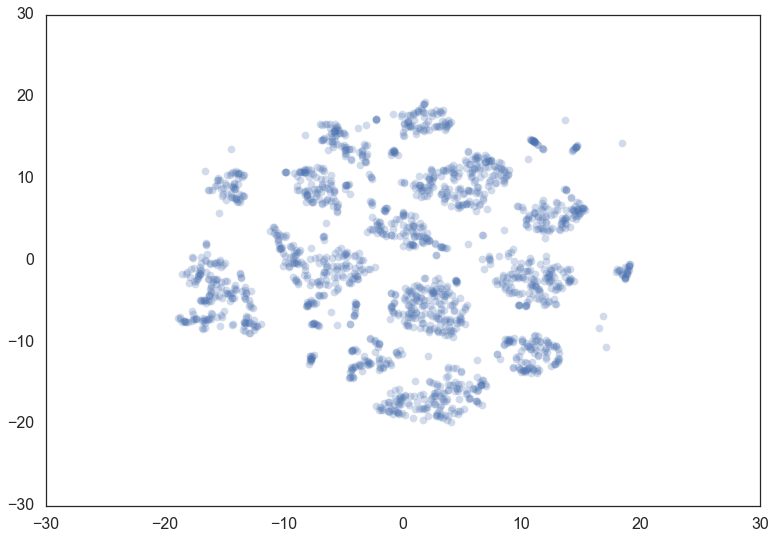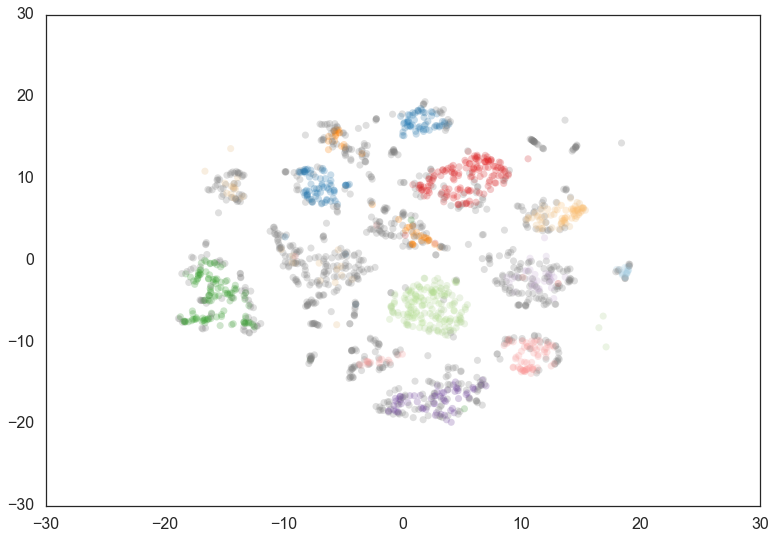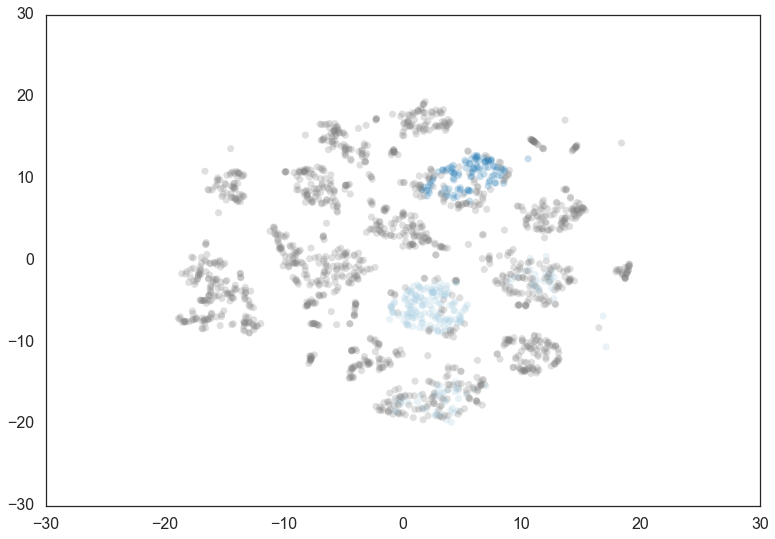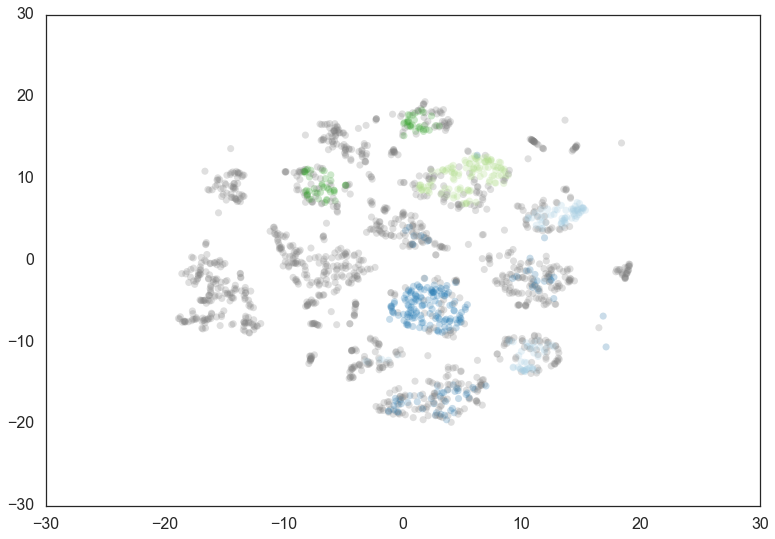# Parameter Selection for HDBSCAN*¶

While the HDBSCAN class has a large number of parameters that can be set on initialization, in practice there are a very small number of parameters that have significant practical effect on clustering. We will first consider those major parameters, and consider how one may go about choosing them effectively. With that out of the way we’ll look at the remaining parameters and see what their effects are – many just effect performance for various different use cases.

## Selecting `min_cluster_size`¶

The primary parameter to effect the resulting clustering is `min_cluster_size`. Ideally this is a relatively intuitive parameter to select – set it to the smallest size grouping that you wish to consider a cluster. It can have slightly non-obvious effects however. Let’s consider the digits dataset from sklearn. We can project the data into two dimensions to visualize it via t-SNE.

```digits = datasets.load_digits()
data = digits.data
projection = TSNE().fit_transform(data)
plt.scatter(*projection.T, **plot_kwds)
```If we cluster this data in the full 64 dimensional space with HDBSCAN* we can see some effects from varying the `min_cluster_size`.

We start with a `min_cluster_size` of 15.

```clusterer = hdbscan.HDBSCAN(min_cluster_size=15).fit(data)
color_palette = sns.color_palette('Paired', 12)
cluster_colors = [color_palette[x] if x >= 0
else (0.5, 0.5, 0.5)
for x in clusterer.labels_]
cluster_member_colors = [sns.desaturate(x, p) for x, p in
zip(cluster_colors, clusterer.probabilities_)]
plt.scatter(*projection.T, s=50, linewidth=0, c=cluster_member_colors, alpha=0.25)
```Increasing the `min_cluster_size` to 30 reduces the number of clusters, merging some together. This is a result of HDBSCAN* reoptimizing which flat clustering provides greater stability under a slightly different notion of what constitutes a cluster.

```clusterer = hdbscan.HDBSCAN(min_cluster_size=30).fit(data)
color_palette = sns.color_palette('Paired', 12)
cluster_colors = [color_palette[x] if x >= 0
else (0.5, 0.5, 0.5)
for x in clusterer.labels_]
cluster_member_colors = [sns.desaturate(x, p) for x, p in
zip(cluster_colors, clusterer.probabilities_)]
plt.scatter(*projection.T, s=50, linewidth=0, c=cluster_member_colors, alpha=0.25)
```Doubling the `min_cluster_size` again to 60 gives us just two clusters – the really core clusters. This is somewhat as expected, but surely some of the other clusters that we had previously had more than 60 members? Why are they being considered noise? The answer is that HDBSCAN* has a second parameter `min_samples`. The implementation defaults this value (if it is unspecified) to whatever `min_cluster_size` is set to. We can recover some of our original clusters by explicitly providing `min_samples` at the original value of 15.

```clusterer = hdbscan.HDBSCAN(min_cluster_size=60).fit(data)
color_palette = sns.color_palette('Paired', 12)
cluster_colors = [color_palette[x] if x >= 0
else (0.5, 0.5, 0.5)
for x in clusterer.labels_]
cluster_member_colors = [sns.desaturate(x, p) for x, p in
zip(cluster_colors, clusterer.probabilities_)]
plt.scatter(*projection.T, s=50, linewidth=0, c=cluster_member_colors, alpha=0.25)
``````clusterer = hdbscan.HDBSCAN(min_cluster_size=60, min_samples=15).fit(data)
color_palette = sns.color_palette('Paired', 12)
cluster_colors = [color_palette[x] if x >= 0
else (0.5, 0.5, 0.5)
for x in clusterer.labels_]
cluster_member_colors = [sns.desaturate(x, p) for x, p in
zip(cluster_colors, clusterer.probabilities_)]
plt.scatter(*projection.T, s=50, linewidth=0, c=cluster_member_colors, alpha=0.25)
```As you can see this results in us recovering something much closer to our original clustering, only now with some of the smaller clusters pruned out. Thus `min_cluster_size` does behave more closely to our intuitions, but only if we fix `min_samples`. If you wish to explore different `min_cluster_size` settings with a fixed `min_samples` value, especially for larger dataset sizes, you can cache the hard computation, and recompute only the relatively cheap flat cluster extraction using the `memory` parameter, which makes use of `joblib` [link].

## Selecting `min_samples`¶

Since we have seen that `min_samples` clearly has a dramatic effect on clustering, the question becomes: how do we select this parameter? The simplest intuition for what `min_samples` does is provide a measure of how conservative you want you clustering to be. The larger the value of `min_samples` you provide, the more conservative the clustering – more points will be declared as noise, and clusters will be restricted to progressively more dense areas. We can see this in practice by leaving the `min_cluster_size` at 60, but reducing `min_samples` to 1.

```clusterer = hdbscan.HDBSCAN(min_cluster_size=60, min_samples=1).fit(data)
color_palette = sns.color_palette('Paired', 12)
cluster_colors = [color_palette[x] if x >= 0
else (0.5, 0.5, 0.5)
for x in clusterer.labels_]
cluster_member_colors = [sns.desaturate(x, p) for x, p in
zip(cluster_colors, clusterer.probabilities_)]
plt.scatter(*projection.T, s=50, linewidth=0, c=cluster_member_colors, alpha=0.25)
```
```<matplotlib.collections.PathCollection at 0x120978438>
```Now most points are clustered, and there are much fewer noise points. Steadily increasing `min_samples` will, as we saw in the examples above, make the clustering progressivly more conservative, culminating in the example above where `min_samples` was set to 60 and we had only two clusters with most points declared as noise.

## Selecting `alpha`¶

A further parameter that effects the resulting clustering is `alpha`. In practice it is best not to mess with this paramter – ultimately it is part of the `RobustSingleLinkage` code, but flows naturally into HDBSCAN*. If, for some reason, `min_samples` is not providing you what you need, stop, rethink things, and try again with `min_samples`. If you still need to play with another parameter (and you shouldn’t), then you can try setting `alpha`. The `alpha` parameter provides a slightly different approach to determining how conservative the clustering is. By default `alpha` is set to 1.0. Increasing `alpha` will make the clustering more conservative, but on a much tighter scale, as we can see by setting `alpha` to 1.3.

```clusterer = hdbscan.HDBSCAN(min_cluster_size=60, min_samples=15, alpha=1.3).fit(data)
color_palette = sns.color_palette('Paired', 12)
cluster_colors = [color_palette[x] if x >= 0
else (0.5, 0.5, 0.5)
for x in clusterer.labels_]
cluster_member_colors = [sns.desaturate(x, p) for x, p in
zip(cluster_colors, clusterer.probabilities_)]
plt.scatter(*projection.T, s=50, linewidth=0, c=cluster_member_colors, alpha=0.25)
```